Скачать презентацию For Wednesday Read Chapter 18 sections 1

b6228bde4edb8bc3f42684166d4dbaa8.ppt

• Количество слайдов: 38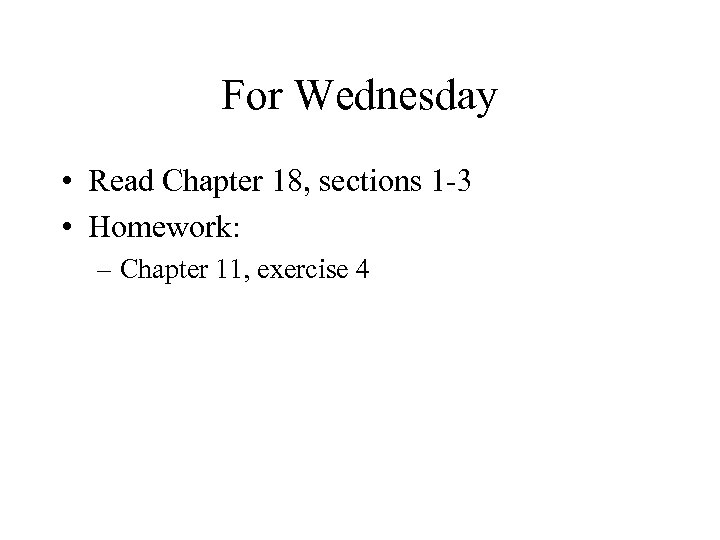For Wednesday • Read Chapter 18, sections 1 3 • Homework: – Chapter 11, exercise 4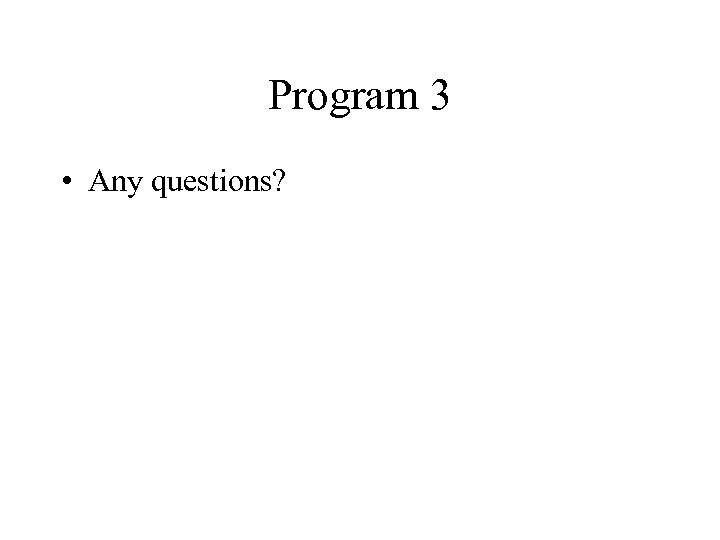Program 3 • Any questions?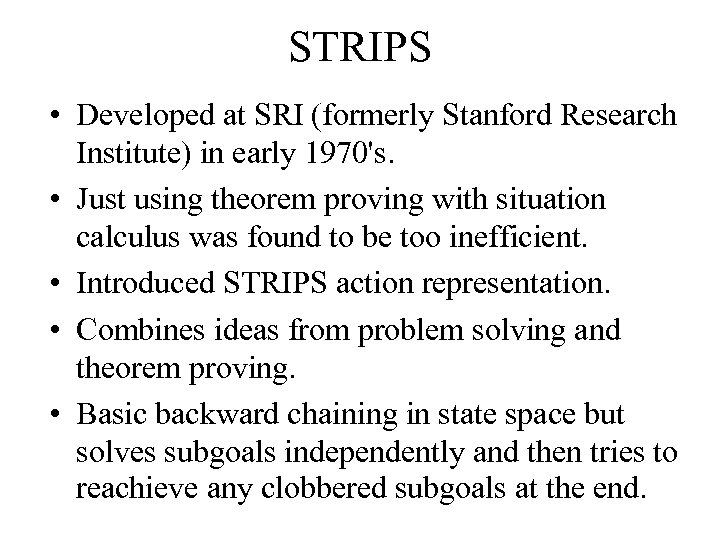STRIPS • Developed at SRI (formerly Stanford Research Institute) in early 1970's. • Just using theorem proving with situation calculus was found to be too inefficient. • Introduced STRIPS action representation. • Combines ideas from problem solving and theorem proving. • Basic backward chaining in state space but solves subgoals independently and then tries to reachieve any clobbered subgoals at the end.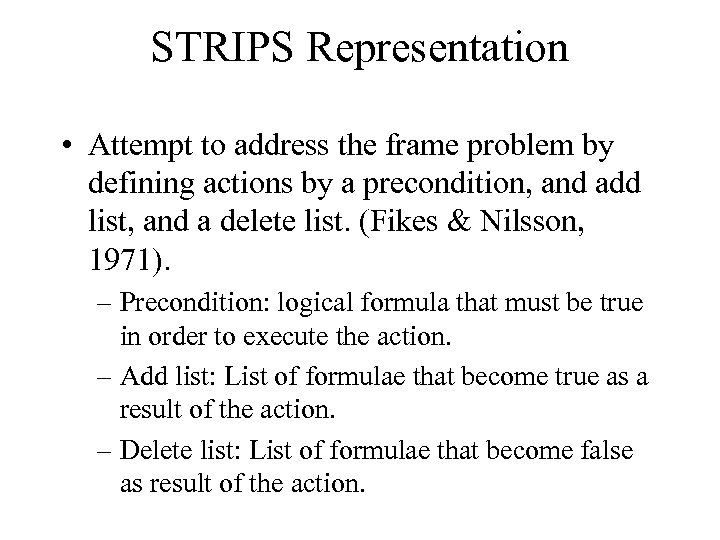STRIPS Representation • Attempt to address the frame problem by defining actions by a precondition, and add list, and a delete list. (Fikes & Nilsson, 1971). – Precondition: logical formula that must be true in order to execute the action. – Add list: List of formulae that become true as a result of the action. – Delete list: List of formulae that become false as result of the action.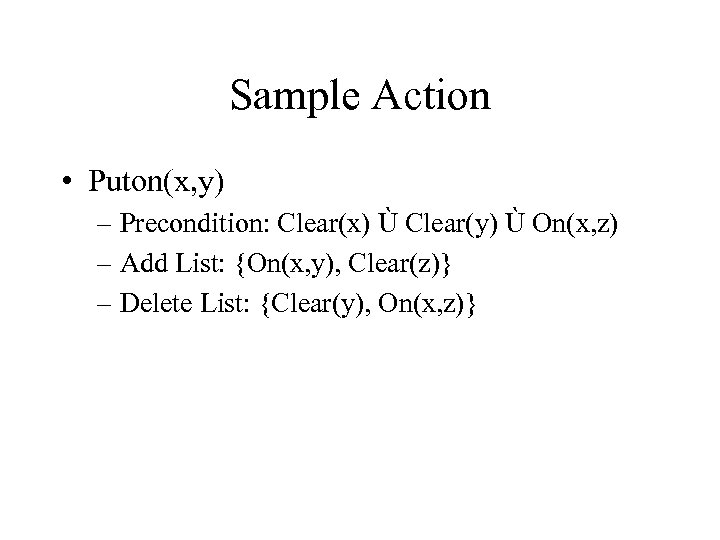Sample Action • Puton(x, y) – Precondition: Clear(x) Ù Clear(y) Ù On(x, z) – Add List: {On(x, y), Clear(z)} – Delete List: {Clear(y), On(x, z)}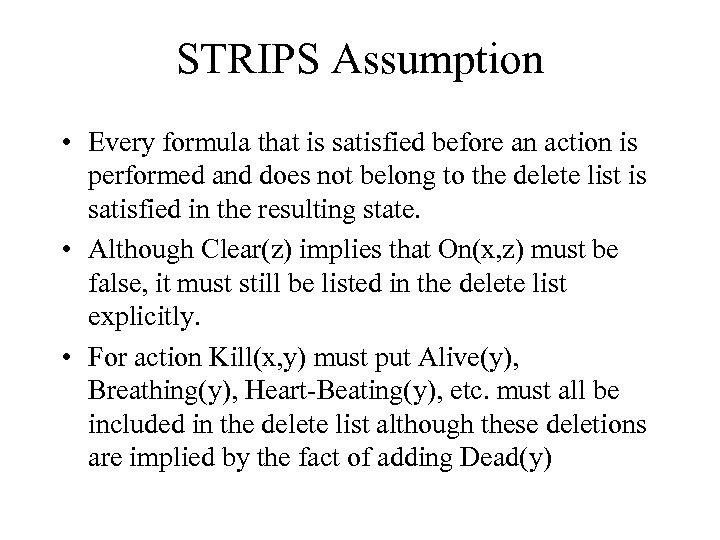STRIPS Assumption • Every formula that is satisfied before an action is performed and does not belong to the delete list is satisfied in the resulting state. • Although Clear(z) implies that On(x, z) must be false, it must still be listed in the delete list explicitly. • For action Kill(x, y) must put Alive(y), Breathing(y), Heart Beating(y), etc. must all be included in the delete list although these deletions are implied by the fact of adding Dead(y)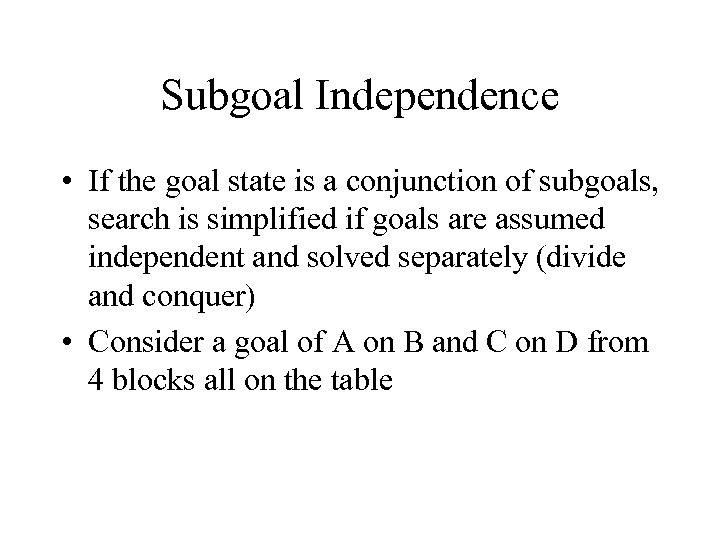Subgoal Independence • If the goal state is a conjunction of subgoals, search is simplified if goals are assumed independent and solved separately (divide and conquer) • Consider a goal of A on B and C on D from 4 blocks all on the table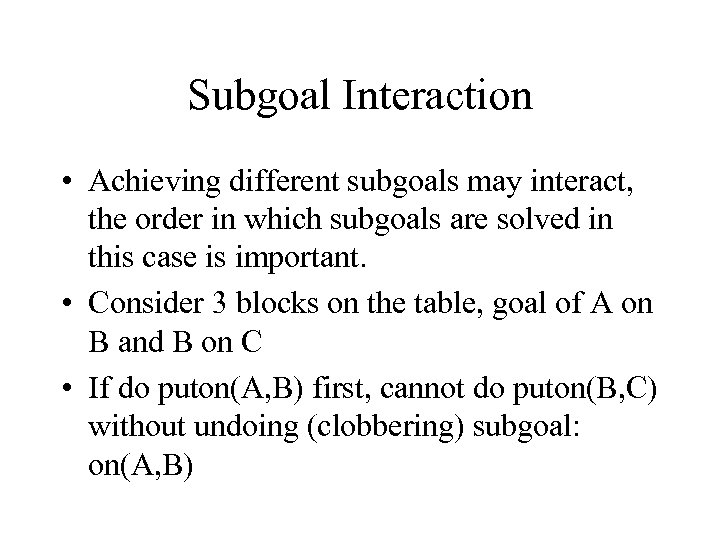Subgoal Interaction • Achieving different subgoals may interact, the order in which subgoals are solved in this case is important. • Consider 3 blocks on the table, goal of A on B and B on C • If do puton(A, B) first, cannot do puton(B, C) without undoing (clobbering) subgoal: on(A, B)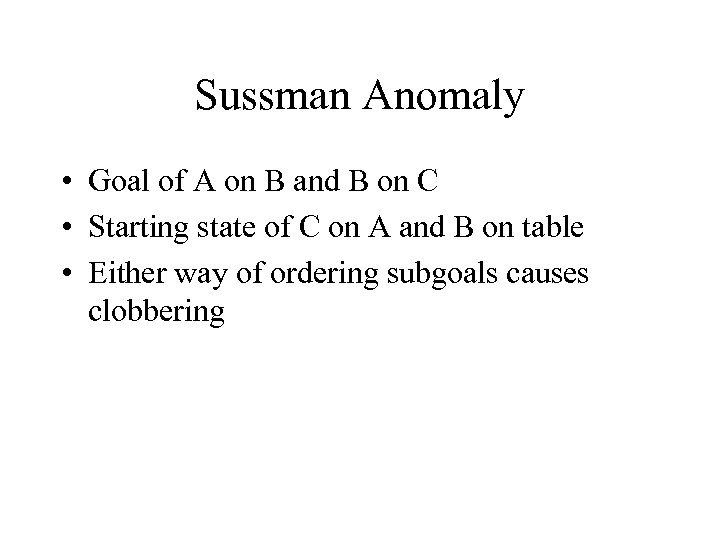Sussman Anomaly • Goal of A on B and B on C • Starting state of C on A and B on table • Either way of ordering subgoals causes clobbering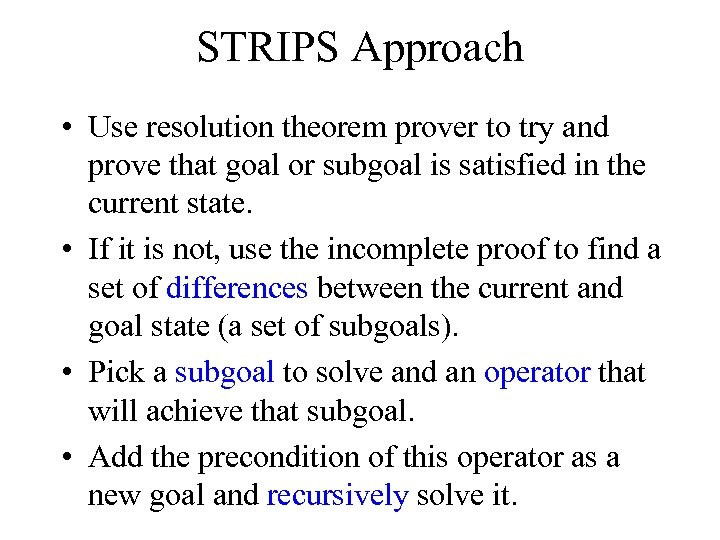STRIPS Approach • Use resolution theorem prover to try and prove that goal or subgoal is satisfied in the current state. • If it is not, use the incomplete proof to find a set of differences between the current and goal state (a set of subgoals). • Pick a subgoal to solve and an operator that will achieve that subgoal. • Add the precondition of this operator as a new goal and recursively solve it.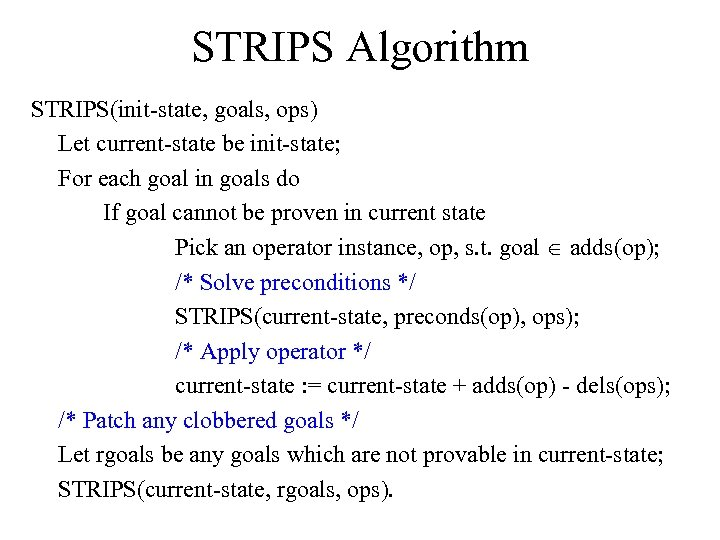STRIPS Algorithm STRIPS(init state, goals, ops) Let current state be init state; For each goal in goals do If goal cannot be proven in current state Pick an operator instance, op, s. t. goal Î adds(op); /* Solve preconditions */ STRIPS(current state, preconds(op), ops); /* Apply operator */ current state : = current state + adds(op) dels(ops); /* Patch any clobbered goals */ Let rgoals be any goals which are not provable in current state; STRIPS(current state, rgoals, ops).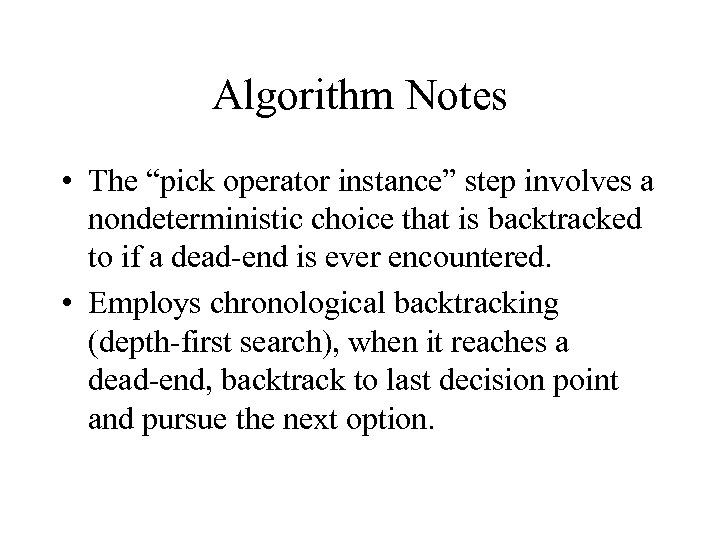Algorithm Notes • The “pick operator instance” step involves a nondeterministic choice that is backtracked to if a dead end is ever encountered. • Employs chronological backtracking (depth first search), when it reaches a dead end, backtrack to last decision point and pursue the next option.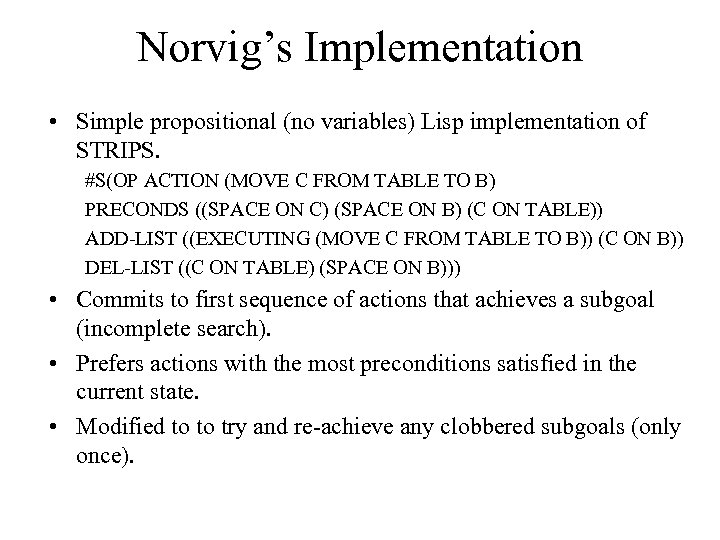Norvig’s Implementation • Simple propositional (no variables) Lisp implementation of STRIPS. #S(OP ACTION (MOVE C FROM TABLE TO B) PRECONDS ((SPACE ON C) (SPACE ON B) (C ON TABLE)) ADD LIST ((EXECUTING (MOVE C FROM TABLE TO B)) (C ON B)) DEL LIST ((C ON TABLE) (SPACE ON B))) • Commits to first sequence of actions that achieves a subgoal (incomplete search). • Prefers actions with the most preconditions satisfied in the current state. • Modified to to try and re achieve any clobbered subgoals (only once).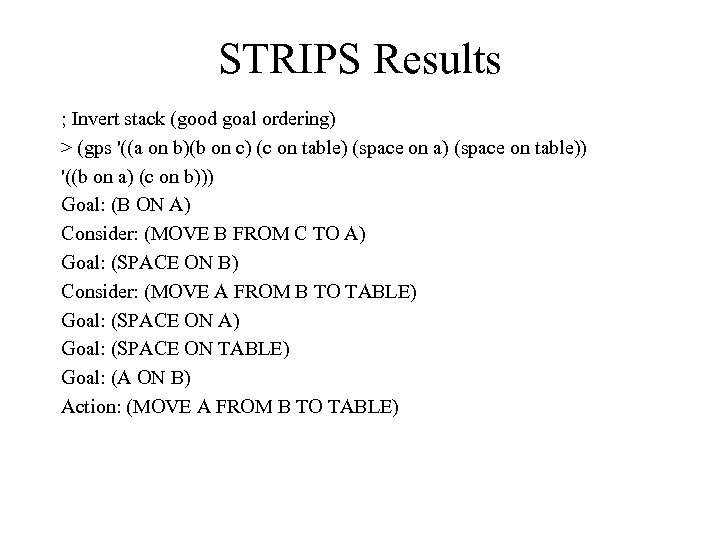STRIPS Results ; Invert stack (good goal ordering) > (gps '((a on b)(b on c) (c on table) (space on a) (space on table)) '((b on a) (c on b))) Goal: (B ON A) Consider: (MOVE B FROM C TO A) Goal: (SPACE ON B) Consider: (MOVE A FROM B TO TABLE) Goal: (SPACE ON A) Goal: (SPACE ON TABLE) Goal: (A ON B) Action: (MOVE A FROM B TO TABLE)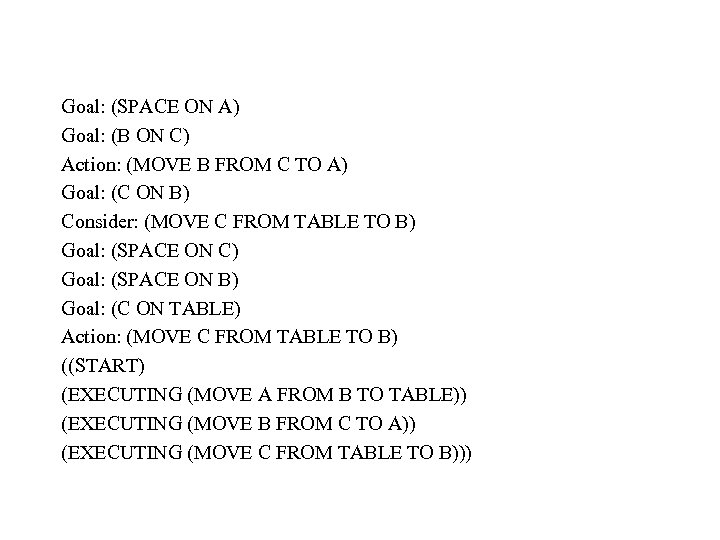Goal: (SPACE ON A) Goal: (B ON C) Action: (MOVE B FROM C TO A) Goal: (C ON B) Consider: (MOVE C FROM TABLE TO B) Goal: (SPACE ON C) Goal: (SPACE ON B) Goal: (C ON TABLE) Action: (MOVE C FROM TABLE TO B) ((START) (EXECUTING (MOVE A FROM B TO TABLE)) (EXECUTING (MOVE B FROM C TO A)) (EXECUTING (MOVE C FROM TABLE TO B)))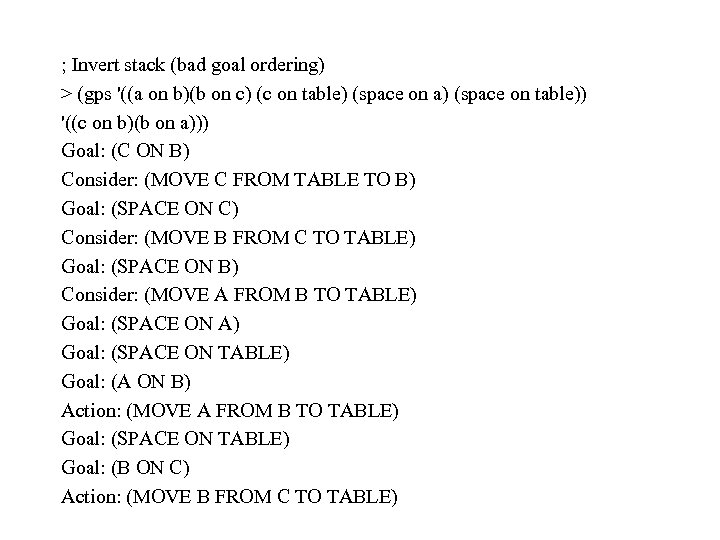; Invert stack (bad goal ordering) > (gps '((a on b)(b on c) (c on table) (space on a) (space on table)) '((c on b)(b on a))) Goal: (C ON B) Consider: (MOVE C FROM TABLE TO B) Goal: (SPACE ON C) Consider: (MOVE B FROM C TO TABLE) Goal: (SPACE ON B) Consider: (MOVE A FROM B TO TABLE) Goal: (SPACE ON A) Goal: (SPACE ON TABLE) Goal: (A ON B) Action: (MOVE A FROM B TO TABLE) Goal: (SPACE ON TABLE) Goal: (B ON C) Action: (MOVE B FROM C TO TABLE)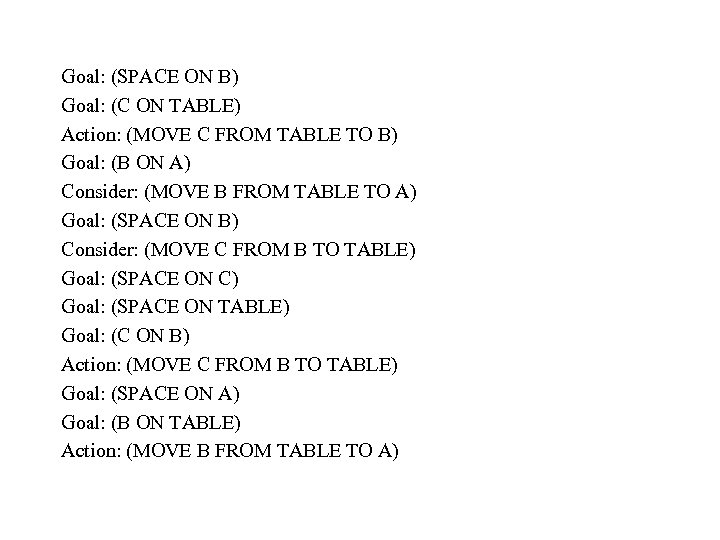Goal: (SPACE ON B) Goal: (C ON TABLE) Action: (MOVE C FROM TABLE TO B) Goal: (B ON A) Consider: (MOVE B FROM TABLE TO A) Goal: (SPACE ON B) Consider: (MOVE C FROM B TO TABLE) Goal: (SPACE ON C) Goal: (SPACE ON TABLE) Goal: (C ON B) Action: (MOVE C FROM B TO TABLE) Goal: (SPACE ON A) Goal: (B ON TABLE) Action: (MOVE B FROM TABLE TO A)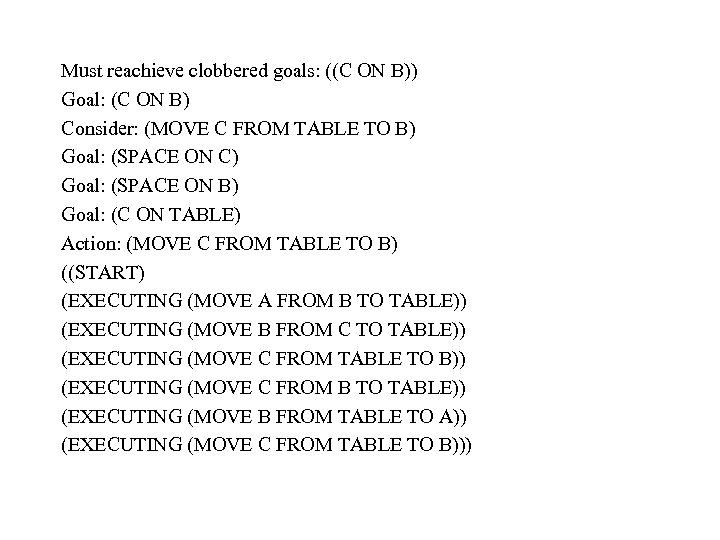Must reachieve clobbered goals: ((C ON B)) Goal: (C ON B) Consider: (MOVE C FROM TABLE TO B) Goal: (SPACE ON C) Goal: (SPACE ON B) Goal: (C ON TABLE) Action: (MOVE C FROM TABLE TO B) ((START) (EXECUTING (MOVE A FROM B TO TABLE)) (EXECUTING (MOVE B FROM C TO TABLE)) (EXECUTING (MOVE C FROM TABLE TO B)) (EXECUTING (MOVE C FROM B TO TABLE)) (EXECUTING (MOVE B FROM TABLE TO A)) (EXECUTING (MOVE C FROM TABLE TO B)))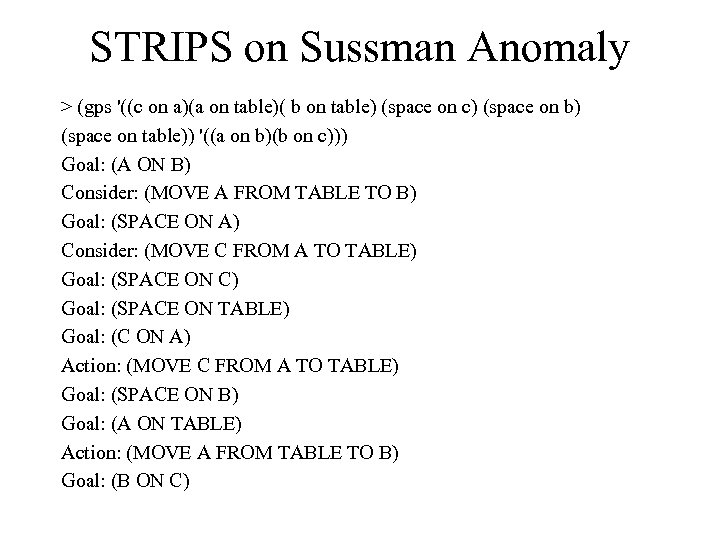STRIPS on Sussman Anomaly > (gps '((c on a)(a on table)( b on table) (space on c) (space on b) (space on table)) '((a on b)(b on c))) Goal: (A ON B) Consider: (MOVE A FROM TABLE TO B) Goal: (SPACE ON A) Consider: (MOVE C FROM A TO TABLE) Goal: (SPACE ON C) Goal: (SPACE ON TABLE) Goal: (C ON A) Action: (MOVE C FROM A TO TABLE) Goal: (SPACE ON B) Goal: (A ON TABLE) Action: (MOVE A FROM TABLE TO B) Goal: (B ON C)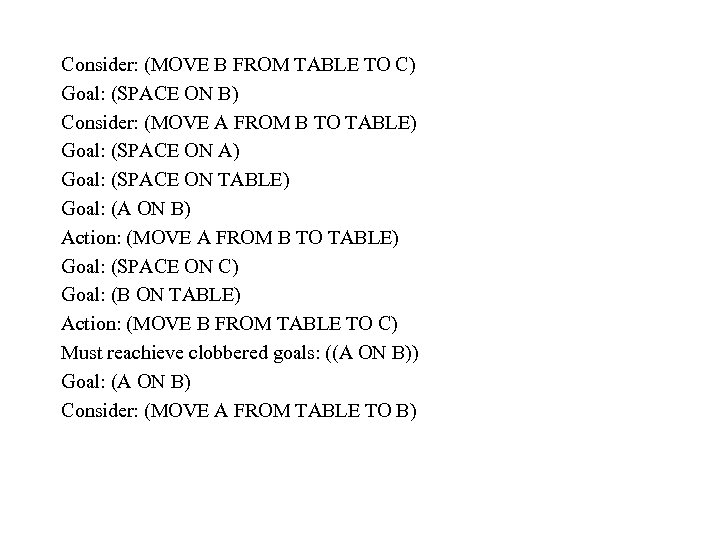Consider: (MOVE B FROM TABLE TO C) Goal: (SPACE ON B) Consider: (MOVE A FROM B TO TABLE) Goal: (SPACE ON A) Goal: (SPACE ON TABLE) Goal: (A ON B) Action: (MOVE A FROM B TO TABLE) Goal: (SPACE ON C) Goal: (B ON TABLE) Action: (MOVE B FROM TABLE TO C) Must reachieve clobbered goals: ((A ON B)) Goal: (A ON B) Consider: (MOVE A FROM TABLE TO B)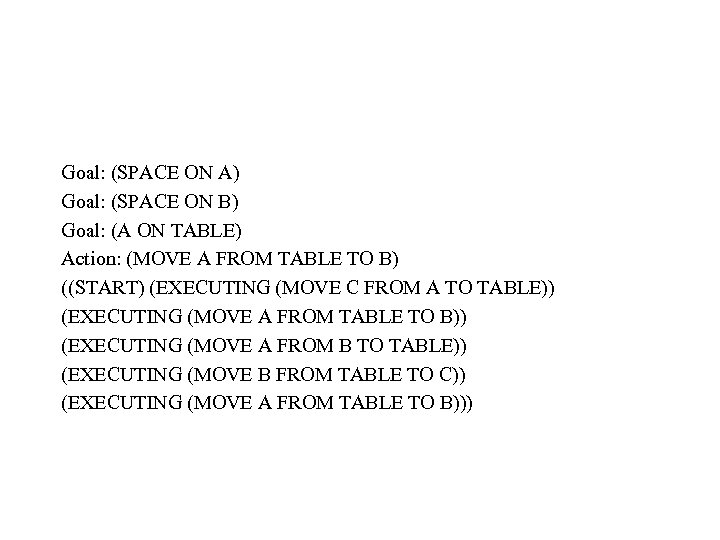Goal: (SPACE ON A) Goal: (SPACE ON B) Goal: (A ON TABLE) Action: (MOVE A FROM TABLE TO B) ((START) (EXECUTING (MOVE C FROM A TO TABLE)) (EXECUTING (MOVE A FROM TABLE TO B)) (EXECUTING (MOVE A FROM B TO TABLE)) (EXECUTING (MOVE B FROM TABLE TO C)) (EXECUTING (MOVE A FROM TABLE TO B)))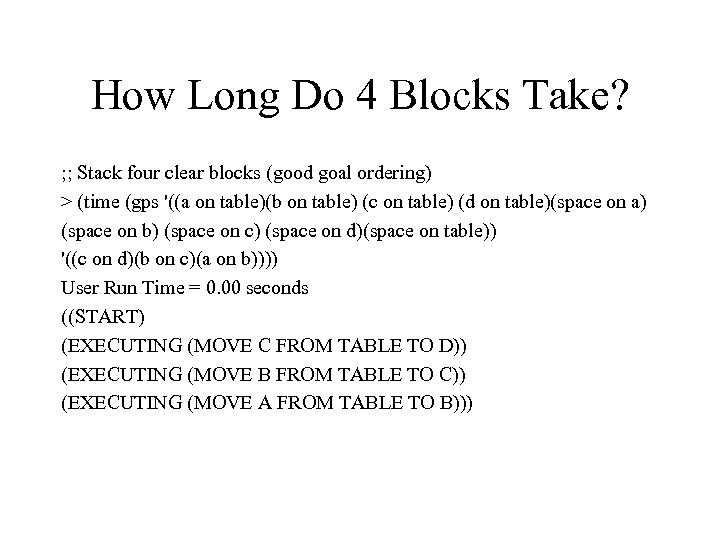How Long Do 4 Blocks Take? ; ; Stack four clear blocks (good goal ordering) > (time (gps '((a on table)(b on table) (c on table) (d on table)(space on a) (space on b) (space on c) (space on d)(space on table)) '((c on d)(b on c)(a on b)))) User Run Time = 0. 00 seconds ((START) (EXECUTING (MOVE C FROM TABLE TO D)) (EXECUTING (MOVE B FROM TABLE TO C)) (EXECUTING (MOVE A FROM TABLE TO B)))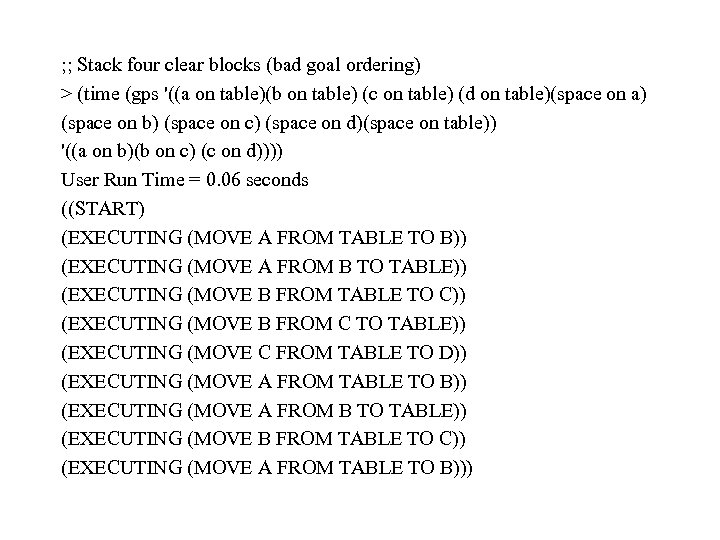; ; Stack four clear blocks (bad goal ordering) > (time (gps '((a on table)(b on table) (c on table) (d on table)(space on a) (space on b) (space on c) (space on d)(space on table)) '((a on b)(b on c) (c on d)))) User Run Time = 0. 06 seconds ((START) (EXECUTING (MOVE A FROM TABLE TO B)) (EXECUTING (MOVE A FROM B TO TABLE)) (EXECUTING (MOVE B FROM TABLE TO C)) (EXECUTING (MOVE B FROM C TO TABLE)) (EXECUTING (MOVE C FROM TABLE TO D)) (EXECUTING (MOVE A FROM TABLE TO B)) (EXECUTING (MOVE A FROM B TO TABLE)) (EXECUTING (MOVE B FROM TABLE TO C)) (EXECUTING (MOVE A FROM TABLE TO B)))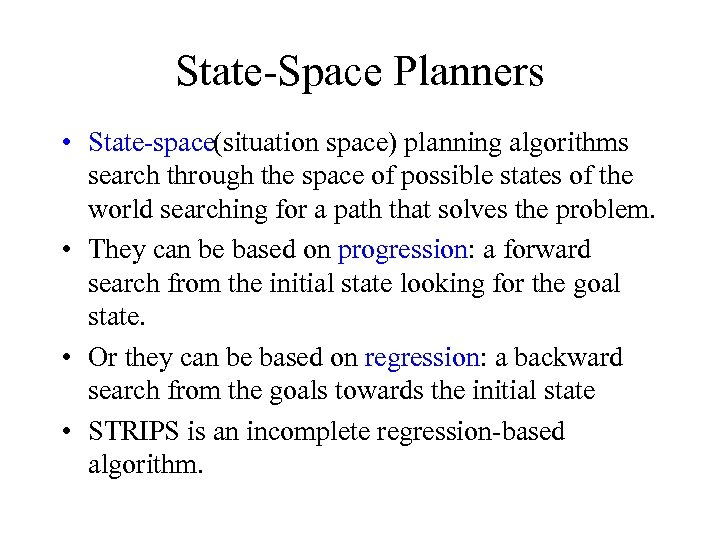State Space Planners • State space(situation space) planning algorithms search through the space of possible states of the world searching for a path that solves the problem. • They can be based on progression: a forward search from the initial state looking for the goal state. • Or they can be based on regression: a backward search from the goals towards the initial state • STRIPS is an incomplete regression based algorithm.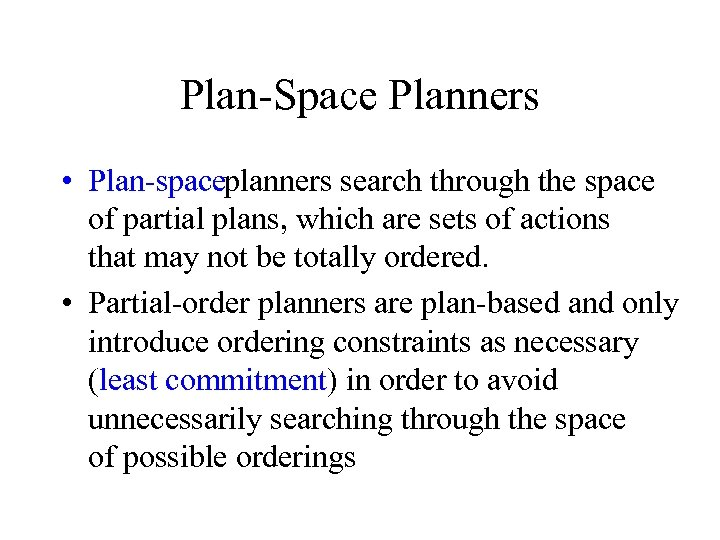Plan Space Planners • Plan spaceplanners search through the space of partial plans, which are sets of actions that may not be totally ordered. • Partial order planners are plan based and only introduce ordering constraints as necessary (least commitment) in order to avoid unnecessarily searching through the space of possible orderings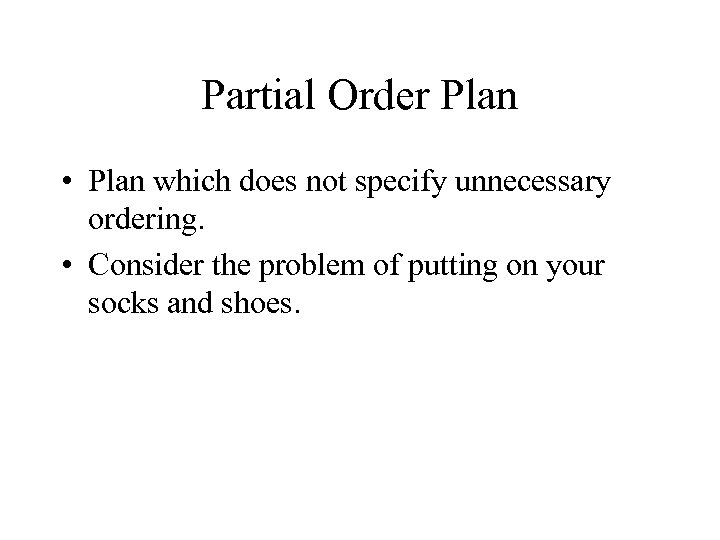Partial Order Plan • Plan which does not specify unnecessary ordering. • Consider the problem of putting on your socks and shoes.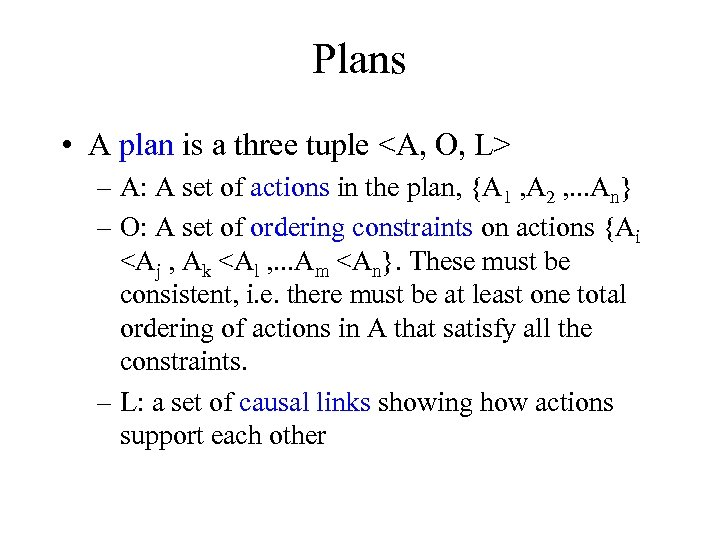Plans • A plan is a three tuple – A: A set of actions in the plan, {A 1 , A 2 , . . . An} – O: A set of ordering constraints on actions {Ai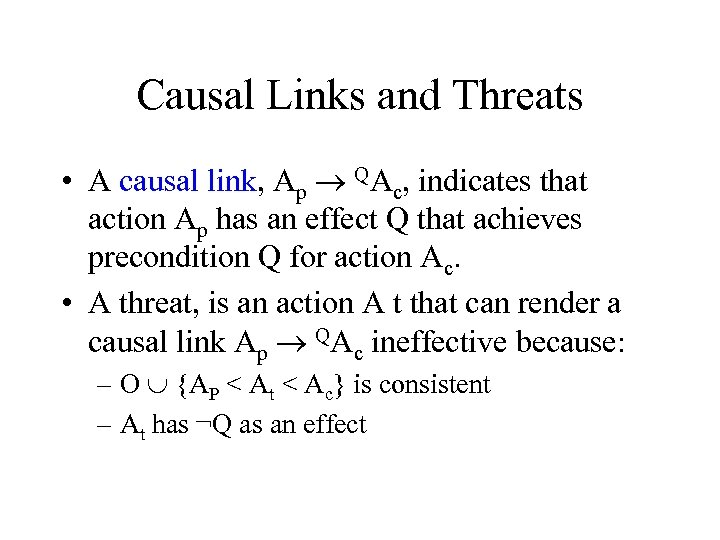Causal Links and Threats • A causal link, Ap ® QAc, indicates that action Ap has an effect Q that achieves precondition Q for action Ac. • A threat, is an action A t that can render a causal link Ap ® QAc ineffective because: – O È {AP < At < Ac} is consistent – At has ¬Q as an effect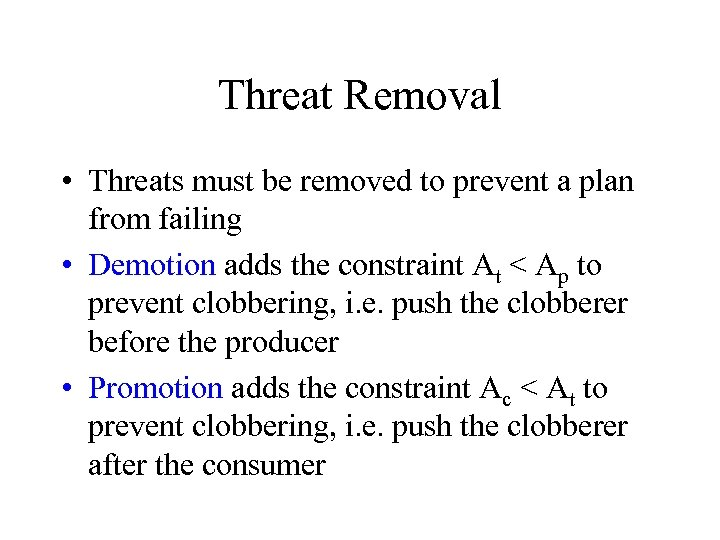Threat Removal • Threats must be removed to prevent a plan from failing • Demotion adds the constraint At < Ap to prevent clobbering, i. e. push the clobberer before the producer • Promotion adds the constraint Ac < At to prevent clobbering, i. e. push the clobberer after the consumer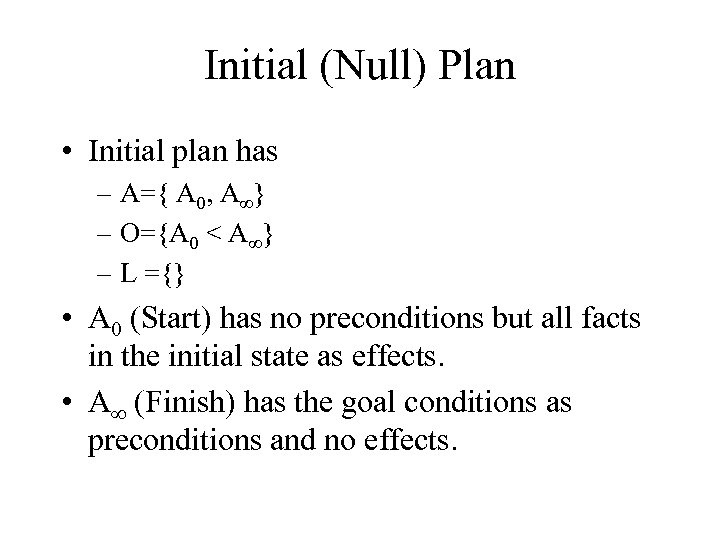Initial (Null) Plan • Initial plan has – A={ A 0, A¥} – O={A 0 < A¥} – L ={} • A 0 (Start) has no preconditions but all facts in the initial state as effects. • A¥ (Finish) has the goal conditions as preconditions and no effects.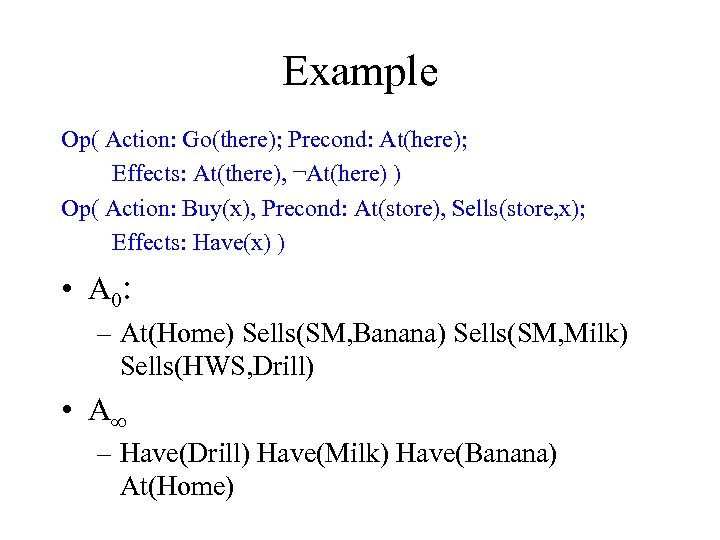Example Op( Action: Go(there); Precond: At(here); Effects: At(there), ¬At(here) ) Op( Action: Buy(x), Precond: At(store), Sells(store, x); Effects: Have(x) ) • A 0: – At(Home) Sells(SM, Banana) Sells(SM, Milk) Sells(HWS, Drill) • A¥ – Have(Drill) Have(Milk) Have(Banana) At(Home)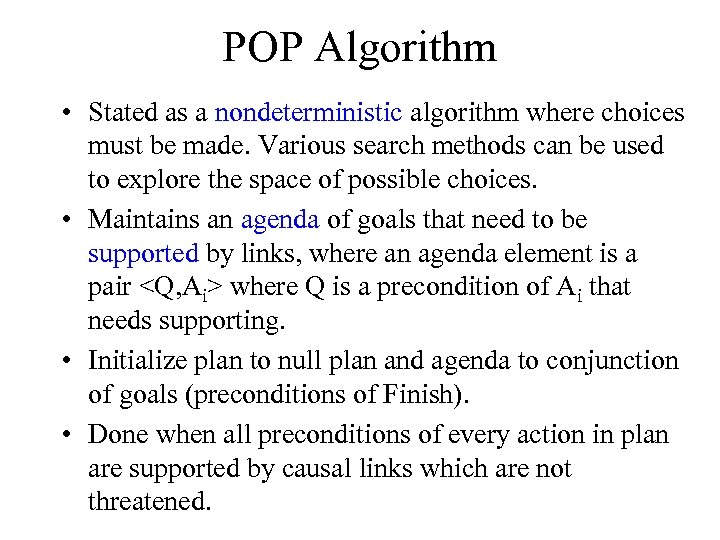POP Algorithm • Stated as a nondeterministic algorithm where choices must be made. Various search methods can be used to explore the space of possible choices. • Maintains an agenda of goals that need to be supported by links, where an agenda element is a pair where Q is a precondition of Ai that needs supporting. • Initialize plan to null plan and agenda to conjunction of goals (preconditions of Finish). • Done when all preconditions of every action in plan are supported by causal links which are not threatened.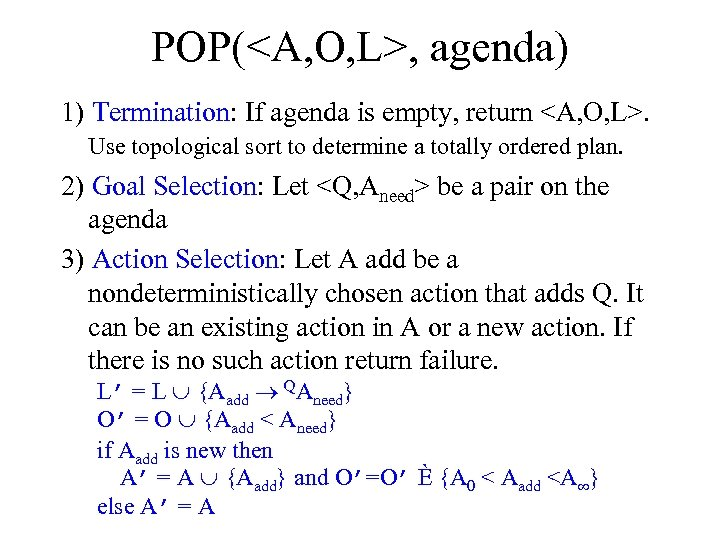POP(, agenda) 1) Termination: If agenda is empty, return . Use topological sort to determine a totally ordered plan. 2) Goal Selection: Let be a pair on the agenda 3) Action Selection: Let A add be a nondeterministically chosen action that adds Q. It can be an existing action in A or a new action. If there is no such action return failure. L’ = L È {Aadd ® QAneed} O’ = O È {Aadd < Aneed} if Aadd is new then A’ = A È {Aadd} and O’=O’ È {A 0 < Aadd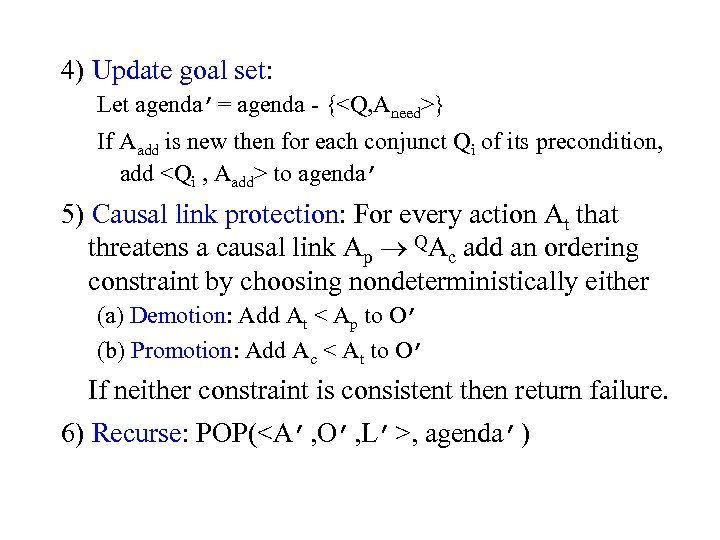4) Update goal set: Let agenda’= agenda {} If Aadd is new then for each conjunct Qi of its precondition, add to agenda’ 5) Causal link protection: For every action At that threatens a causal link Ap ® QAc add an ordering constraint by choosing nondeterministically either (a) Demotion: Add At < Ap to O’ (b) Promotion: Add Ac < At to O’ If neither constraint is consistent then return failure. 6) Recurse: POP(, agenda’)Example Op( Action: Go(there); Precond: At(here); Effects: At(there), ¬At(here) ) Op( Action: Buy(x), Precond: At(store), Sells(store, x); Effects: Have(x) ) • A 0: – At(Home) Sells(SM, Banana) Sells(SM, Milk) Sells(HWS, Drill) • A¥ – Have(Drill) Have(Milk) Have(Banana) At(Home)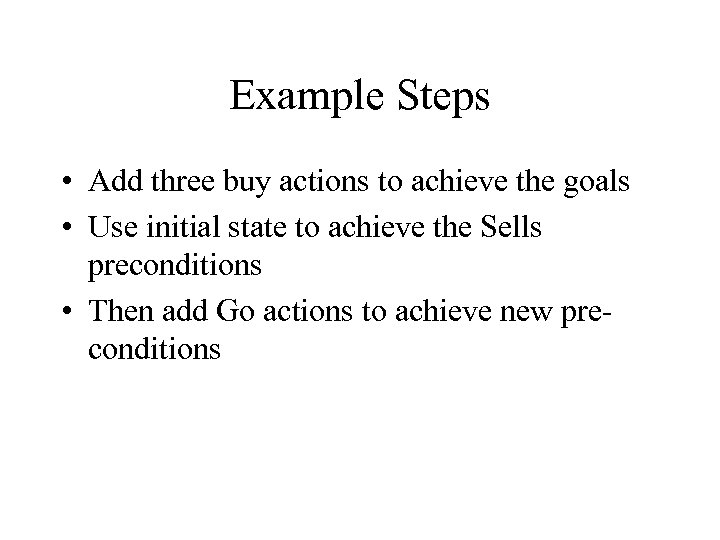Example Steps • Add three buy actions to achieve the goals • Use initial state to achieve the Sells preconditions • Then add Go actions to achieve new pre conditions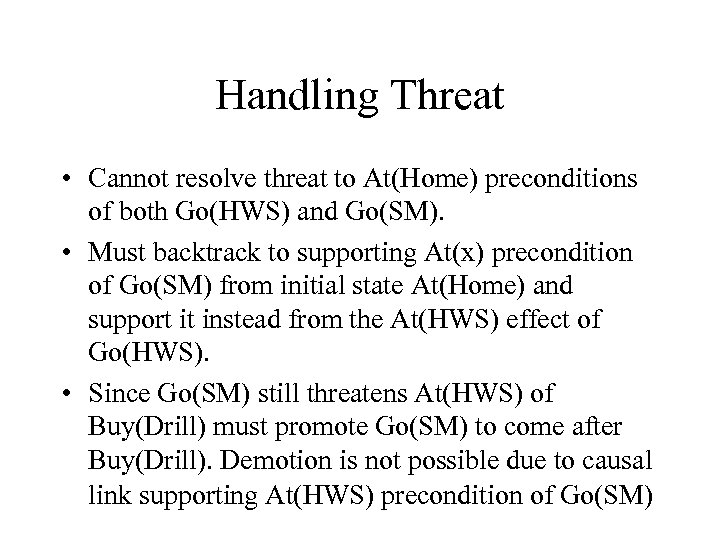Handling Threat • Cannot resolve threat to At(Home) preconditions of both Go(HWS) and Go(SM). • Must backtrack to supporting At(x) precondition of Go(SM) from initial state At(Home) and support it instead from the At(HWS) effect of Go(HWS). • Since Go(SM) still threatens At(HWS) of Buy(Drill) must promote Go(SM) to come after Buy(Drill). Demotion is not possible due to causal link supporting At(HWS) precondition of Go(SM)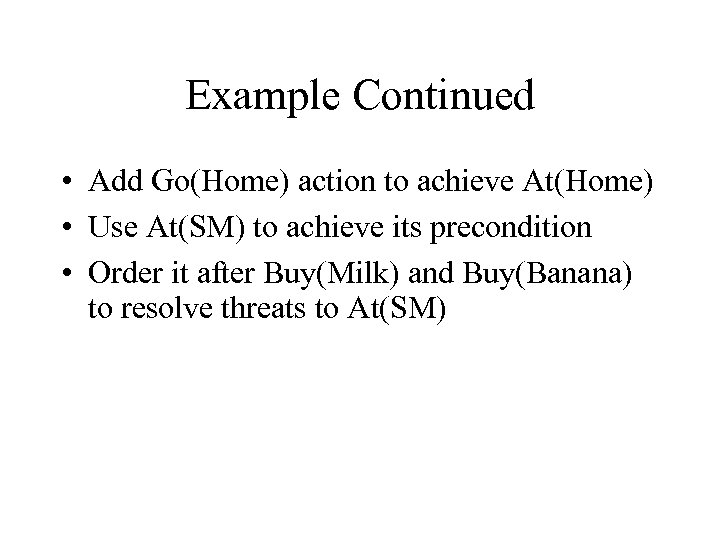Example Continued • Add Go(Home) action to achieve At(Home) • Use At(SM) to achieve its precondition • Order it after Buy(Milk) and Buy(Banana) to resolve threats to At(SM)